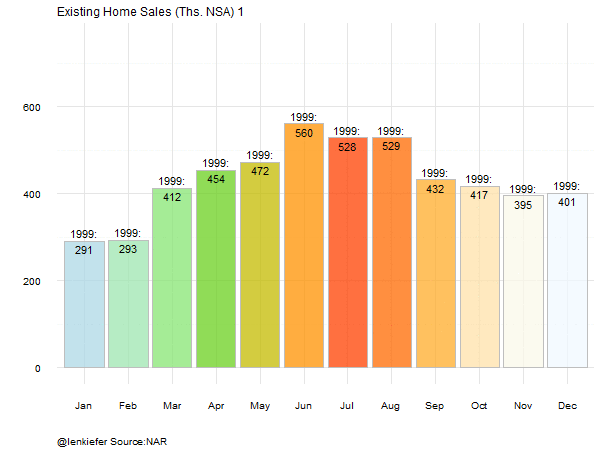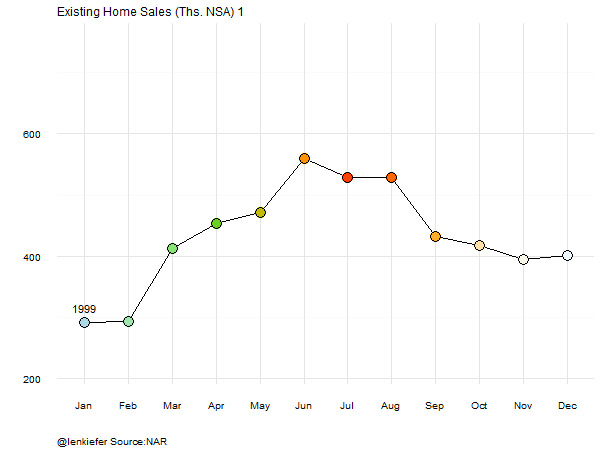# Improving R animated GIFs with tweenr

RECENTLY I HAVE MADE several animated GIFs, primarily using the animation package. These gifs usually work pretty well, coming out something like this (code here).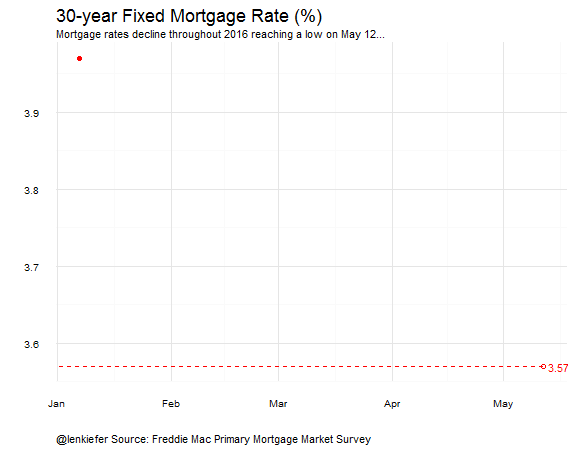Unfortunately, these gifs tend to come out rather choppy. I tried to get around that by using variable length sequences to alter the timing of each frame. My primitive approach involves loops and business like this:

{% highlight r for (i in c(seq(1,365,6),seq(378,716,13),seq(1145,1574,26),seq(1587,2003,13),seq(2016,2185,6),2196) ) { {% endhighlight

What I was trying to achieve with this clumsy sequence was an easing effect. Here’s a handy tipsheet on easing functions with interactive examples. From the link above we have the following:

Easing functions specify the rate of change of a parameter over time. Objects in real life don’t just start and stop instantly, and almost never move at a constant speed. When we open a drawer, we first move it quickly, and slow it down as it comes out. Drop something on the floor, and it will first accelerate downwards, and then bounce back up after hitting the floor. -easings.net

Adding an easing effect would smooth out the animation and make it much more natural. Transitions and easing have been implemented in d3 for some time and have been used to great effect (just look at em!).

I’ve played around with d3 a bit and it’s truly magical. Unfortunately, d3 is not really a data analysis tool and that’s what I do. Wouldn’t it be nice to be able to generate nice smooth transitions in R without having to code up in d3?

## C’mon internet

Fortunately, the internet does what it does and brought me to someone who had solved my exact problem. Turns out that thomasp85 has built a R package called tweenr that does the hard work of interpolating (“tweening”) between frames to generate an easing effect.

It took some experimenting to generate the effects I wanted (and I’m still exploring), but I wanted to write up my approach. There’s not a lot of examples with the tweenr package, so these examples might be helpful for others who want to create their own awesome animated GIFs with R.

Today we’ll start off with just one example, with more to come later.

# House Price GIF

In this example we’ll go from the GIF on the left to the GIF on the right: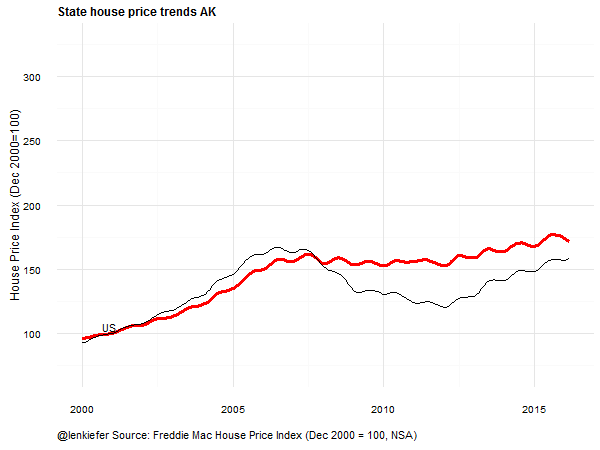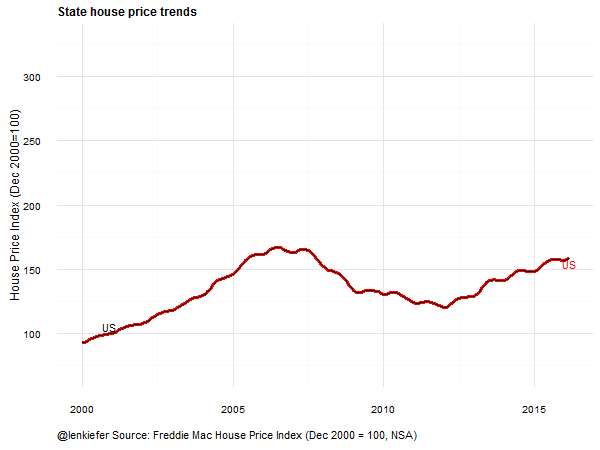We’ll be using the same house price data from my visual meditations series.

### Set up

This code will load the libraries we need and set up our data. You can get the text file with house prices called fmhpi.txt here.

{% highlight r

# Set up libraries

library(“ggplot2”) library(“scales”) library(‘ggthemes’) library(“plyr”) library(“dplyr”) library(“data.table”) library(“animation”) library(“gganimate”) library(“tweenr”)

### Prepare data

statedata <- fread(“fmhpi.txt”) #house price data statedata$date<-as.Date(statedata$date, format=“%m/%d/%Y”) #convert date to Date format

sdata<-statedata[year>1999, list(date,year,state,hpi)] #truncate data to just year>1999 and keep only columns we need

#variables we don’t want to interpolate need to be factors-not character-or tweenr will try to interpolate sdata$year<-as.factor(sdata$year) sdata$state<-as.factor(sdata$state) {% endhighlight

## Animation with no easing

First, let’s go over the animation without easing. I’m going to use the gganimate package, which I’ve been avoiding using because I’m a data masochist and love writing loops. By using gganimate, we can crate the GIF with less code. We lose some control, but gain some efficiency.

We’re going to make a fairly simple data visualization. We’re going to plot the Freddie Mac House Price Index (FMHPI) from January 2000 through March 2016, comparing the national index to each of the 50 states and the District of Columbia.

We’ll be a little inefficient here and append back on the house price index for the national level to our state data set. We could probably avoid this step, but I couldn’t get it to work with gganimate. Then we’ll plot a GIF for each state comparing the state index to the national index.

The transitions will be choppy.

Note 04/13/2017: This post has been updated to correct for a mysterious data frame called dt3, which was not created but called for in the original code. I have replaced dt3 with a data frame that does exist. Now the code should work. Thanks to @butterflyology for pointing this out @dataandme for sharing this post and letting others find it almost a year later.

{% highlight r

# we’ll use the US average as referecne line

data.us<-sdata[state==“US”,] data.us<-dplyr::rename(data.us, ushpi=hpi) #rename hpi column US HPI data.us$state=NULL #drop state column #Create data set for the no easing animation data.noease<-merge(sdata,data.us,by=“date”) #create static data set #animate through gganimate p<- ggplot(data=data.noease,aes(x=date,y=hpi,frame=state,label=state))+ geom_line(aes(cumulative=F),color=“red”,size=1.2)+ geom_line(aes(x=date,y=ushpi,frame=state),color=“black”)+ theme_minimal()+ theme(plot.title=element_text(face=“bold”,size=12))+ # Orginally this code was called, but dt3 doesn’t exist so it won’t work #geom_text(data=dt3[date==“2000-12-01”],aes(x=date,y=ushpi,label=“US”),nudge_y=5, color=“black”)+ # replace with this and it will work: geom_text(data=data.us[date=="2000-12-01"],inherit.aes=F,aes(x=date,y=ushpi,label="US"),nudge_y=5, color="black")+  theme(plot.caption=element_text(hjust=0))+ labs(y=“House Price Index (Dec 2000=100)”, x=“”, caption=“@lenkiefer Source: Freddie Mac House Price Index (Dec 2000 = 100, NSA)“, #subtitle=paste(as.character(statedata[year==yy & month==mm & state==“US”]$date,format=“%b-%Y”)), title=“State house price trends”)

gg_animate(p, “ex1_notween.gif”, title_frame =T, ani.width = 600, ani.height = 450, interval=0.2) {% endhighlight

Run it and you get:## Using tweenr

The GIF above is not bad, but wouldn’t it be nice to have a smooth transition? Fortunately, the tweenr package will do all the hard word and create intermediate frames between transitions to give a smoother animation effect.

tweenr is a great package, but it’s relatively new and doesn’t have a lot of examples I could find. So it took a little tinkering to get the effects I want. The approach I settled on was to use the tween_states function. To use this function you need a sequence of data sets, each one containing a state you want to transition between.

The code below gets us to the tween_states function:

{% highlight r

states<-unique(sdata[state!=“US”]$state) #get a list of states excluding the US #add the “US” at the top and the bottomw of the list of states states2<-factor(c(as.character(“US”),as.character(states),“US”)) # function to create list of data sets from our data (by state) myf<-function(mystate){as.data.frame(sdata[state==mystate])} # use lapply to generate the list of data sets: my.list2<-lapply(states2,myf) # Apply tweenr: tf <- tween_states(my.list2, tweenlength= 2, statelength=3, ease=rep(‘cubic-in-out’,51),nframes=300) {% endhighlight ### Looking at the tweenr function From the tweenr documentation we have: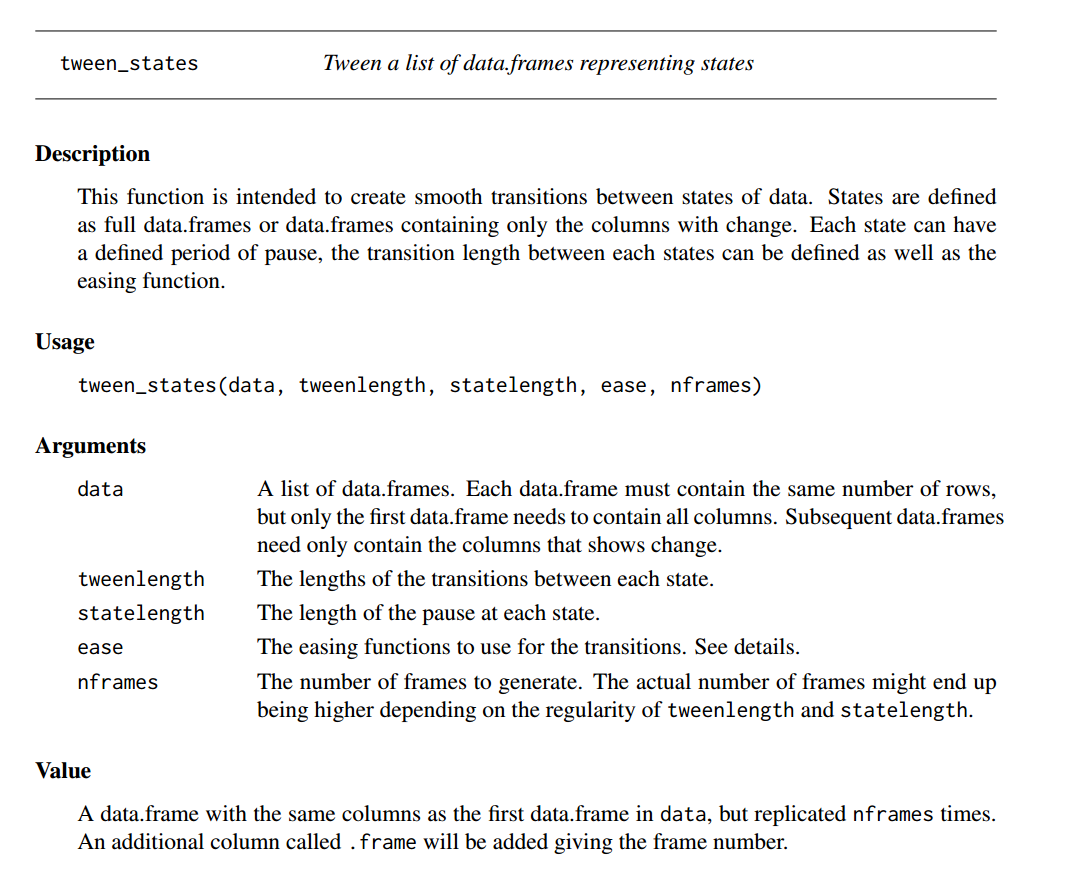I’ve created 53 datasets corresponding to the 50 states plus the District of Columbia, padded with the US at the top and bottom. Then I used the lapply function along with a small user defined function to create the list of data.frames (tweenr doesn’t like data.tables) to feed into the tween_states function. I set the parameters tweenlength to 2 and statelength to 3, meaning we will spend 32 as much time pausing between animations and transitioning between them. I chose the “cubic-in-out” easing function because I like how it looks. Tweenr lets you choose from many different easing functions. I’m looking forward to try some others out. {% highlight r tf <- tween_states(my.list2, tweenlength= 2, statelength=3, ease=rep(‘cubic-in-out’,51),nframes=300) dtf<-data.table(tf) # Make tweenr output a data table dtus<-sdata[state==“US”,] #pull out just the “US dtus<-dplyr::rename(dtus, ushpi=hpi) #rename hpi column US HPI dtus$state=NULL #drop state column

#Create data set for easing animation data.ease<-merge(dtf,dtus,by=“date”) #merge back US as its own variable

# create the animation

p<- ggplot(data=dtf2,aes(x=date,y=hpi,frame=.frame,label=state))+ # setting cumluative=T would leave a trail of lines, which I don’t want here: geom_line(aes(cumulative=F),color=“red”,size=1.2)+ # add us reference line: geom_line(aes(x=date,y=ushpi,frame=.frame),color=“black”)+ geom_text(data=dtf[date==max(dtf\$date)],nudge_y=-5, color=“red”)+ theme_minimal()+ theme(plot.title=element_text(face=“bold”,size=12))+ geom_text(data=dtf2[date==“2000-12-01”],aes(x=date,y=ushpi,frame=.frame,label=“US”),nudge_y=5, color=“black”)+ theme(plot.caption=element_text(hjust=0))+ labs(y=“House Price Index (Dec 2000=100)”, x=“”, caption=“@lenkiefer Source: Freddie Mac House Price Index (Dec 2000 = 100, NSA)“, title=“State house price trends”)

#use gganimate to make the GIF: gganimate(p, “ex2_tween.gif”, title_frame = F, ani.width = 600, ani.height = 450, interval=0.05) {% endhighlight

Run it and you get this result: